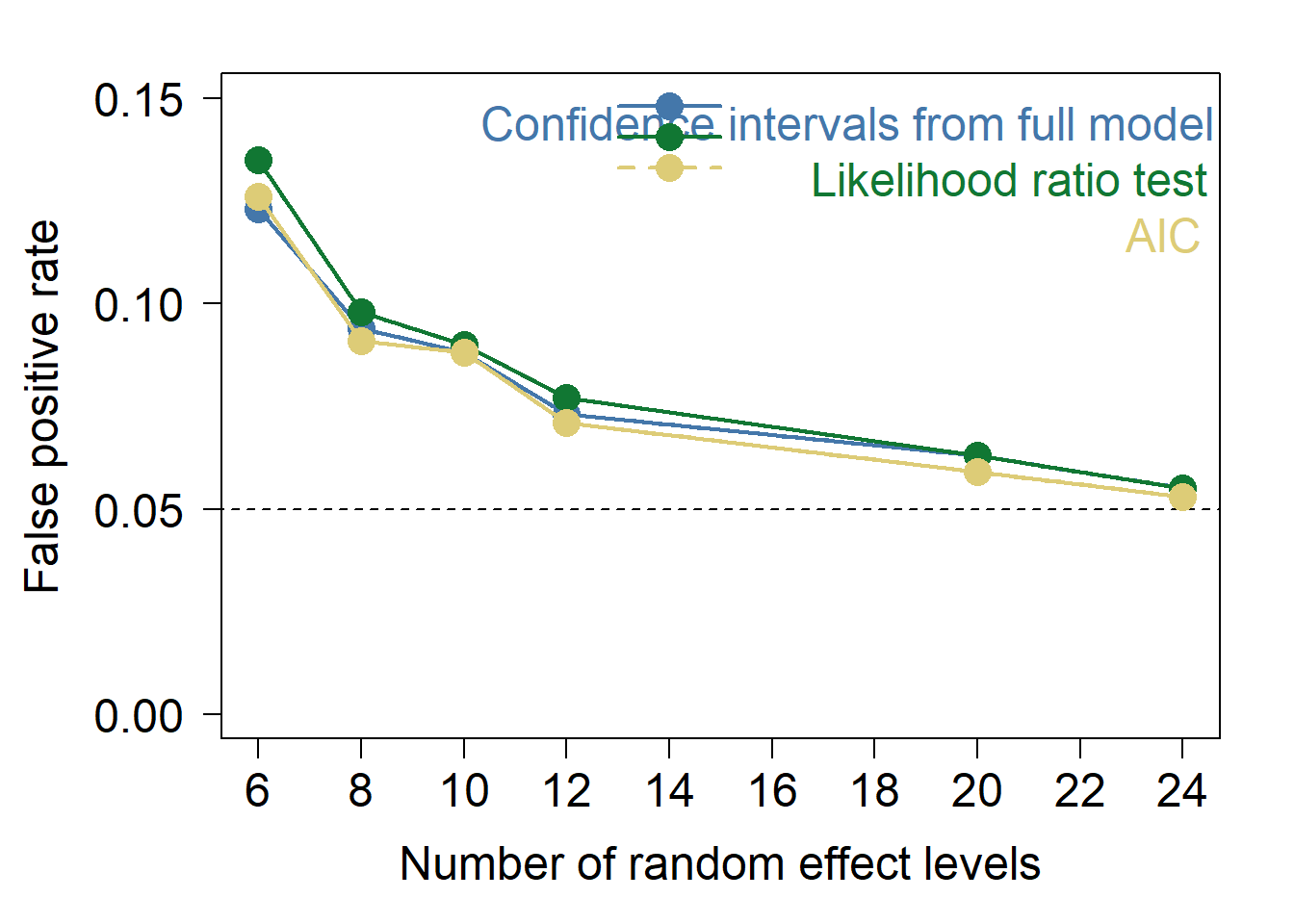Peril 2: Pseudoreplication

library(nlme)
library(lme4)
library(lmerTest)

Define functions - we use this function to produce our plots later

fp_check<-function(x,thresh,dir){
if(dir==1){return(sum(abs(x)<thresh))}
if(dir==2){return(sum(abs(x)>thresh))}
}

Here we define the number of families to use and the number of samples per family. These numbers are chosen to consistently produce a total sample size of 600.

#Define numbers of clones - 7 different number of random effect levels
families<-c(6,8,10,12,20,24)
#Define number of samples per clone - change these to keep the overall sample size the same
perfamily<-c(100,75,60,40,30,25)

Here we set up a number of empty matrices to store the results of the simulations

#Create empty matrices to store results
cis<-matrix(0,1000,nc=6)
ltests<-matrix(0,1000,nc=6)
AICcheck.ML<-matrix(0,nr=1000,nc=6)
cis.lmer<-matrix(0,1000,nc=6)
ltests.lmer<-matrix(0,1000,nc=6)
AICcheck.lmer.ML<-matrix(0,nr=1000,nc=6)
pvals.glm<-matrix(0,nr=1000,nc=6)

Here we conduct the simulations

We first loop over the number of random effect levels (6 in total). Then we conduct 1000 simulations for each number of random effect levels, simulating data as described in the main text of the paper and fitting the model:

y ~ treatment + (1|family)

using both nlme and lme4 as described

NB. We are suppressing the warning messages from model fitting from being printed here for presentation purposes. We would recommend re-running after deleting warning=FALSE and message=FALSE from the line below this to see what warnings arise and when they do if you are interested.

#start loop over different number of random effect levels
for(k in 1:6){

#start loop over different iterations of the simulations
for(i in 1:1000){
#Generate clone factor
family<-gl(families[k],perfamily[k])
#Generate treatment factor
treat<-gl(2,length(family)/2)
#Simulate group-level means for response variable
y.mean<-rep(rnorm(families[k],0,1),each=perfamily[k])
#Simulate values of response variable for each observation
y.obs<-rnorm(length(family),y.mean,1)
#Fit treatment model in nlme
m1<-lme(y.obs~treat,random=~1|family, control = lmeControl(opt = "optim"))
#Fit null model in nlme
m2<-lme(y.obs~1,random=~1|family, control = lmeControl(opt = "optim"))
#confidence interval overlaps zero or not
t_ci<-abs(summary(m1)$tTable[2,1])-abs(summary(m1)$tTable[2,2]*1.96)
cis[i,k]<-as.numeric(t_ci>0)
#Fit nlme models with ML rather than REML
m3<-lme(y.obs~treat,random=~1|family,method="ML", control = lmeControl(opt = "optim"))
m4<-update(m3,~.-treat, control = lmeControl(opt = "optim"))
#Calculate p value from likelihood ratio test
ltests[i,k]<-anova(m3,m4)$"p-value" #Calculate delat AIC from mod3/mod4 AICcheck.ML[i,k]<-AIC(m3)-AIC(m4) #Fit models in lme4 with REML m1.lmer<-lme4::lmer(y.obs~treat+(1|family)) m2.lmer<-lme4::lmer(y.obs~1+(1|family)) #confidence interval overlaps zero or not from lme4 t_ci<-abs(summary(m1.lmer)$coefficients[2,1])-abs(summary(m1.lmer)$coefficients[2,2]*1.96) cis.lmer[i,k]<-as.numeric(t_ci>0) #Fit lme4 models with ML rather than REML m3.lmer<-lme4::lmer(y.obs~treat+(1|family),REML=F) m4.lmer<-lme4::lmer(y.obs~1+(1|family),REML=F) #Calculate delta AIC AICcheck.lmer.ML[i,k]<-AIC(m3.lmer)-AIC(m4.lmer) #Calculate p values from likelihood ratio test ltests.lmer[i,k]<-anova(m3.lmer,m4.lmer)$"Pr(>Chisq)"
#Fit glms without controlling for family ID
m1.glm<-glm(y.obs~treat)
m2.glm<-glm(y.obs~1)
#Calculate p values from model anova using GLMs
pvals.glm[i,k]<-anova(m1.glm,m2.glm,test="F")\$"Pr(>F)"
} #end loop over individual simulations
} #end loop over number of families

Produce plot of simulation results

cols<-c("#4477AA", "#117733", "#DDCC77", "#CC6677")

par(mar=c(5,6,2,2))
plot(NULL,ylim=c(0,0.15),xlim=c(6,24),xlab="Number of random effect levels",ylab="",las=1,cex.lab=1.5,cex.axis=1.5,xaxt="n")
axis(side=1,at=seq(6,24,2),cex.axis=1.5)
mtext(text="False positive rate",side=2,line=4.25,cex=1.5)
lines(x=c(-10,50),y=c(0.05,0.05),lty=2)

points(x=families,y=apply(cis.lmer,2,mean),col=cols,pch=16,cex=2)
lines(x=families,y=apply(cis.lmer,2,mean),col=cols,pch=16,lwd=2)
lines(x=families,y=apply(cis,2,mean),col=cols,pch=16,lwd=2,lty=2)
points(x=families,y=apply(cis,2,mean),col=cols,pch=16,cex=2)

points(x=families,y=apply(ltests.lmer,2,fp_check,0.05,1)/1000,col=cols,pch=16,cex=2)
lines(x=families,y=apply(ltests.lmer,2,fp_check,0.05,1)/1000,col=cols,pch=16,lwd=2)
lines(x=families,y=apply(ltests,2,fp_check,0.05,1)/1000,col=cols,pch=16,lwd=2,lty=2)
points(x=families,y=apply(ltests,2,fp_check,0.05,1)/1000,col=cols,pch=16,cex=2)

points(x=families,y=apply(AICcheck.lmer.ML,2,fp_check,2,2)/1000,col=cols,pch=16,cex=2)
lines(x=families,y=apply(AICcheck.lmer.ML,2,fp_check,2,2)/1000,col=cols,pch=16,lwd=2)
lines(x=families,y=apply(AICcheck.ML,2,fp_check,2,2)/1000,col=cols,pch=16,lwd=2,lty=2)
points(x=families,y=apply(AICcheck.ML,2,fp_check,2,2)/1000,col=cols,pch=16,cex=2)